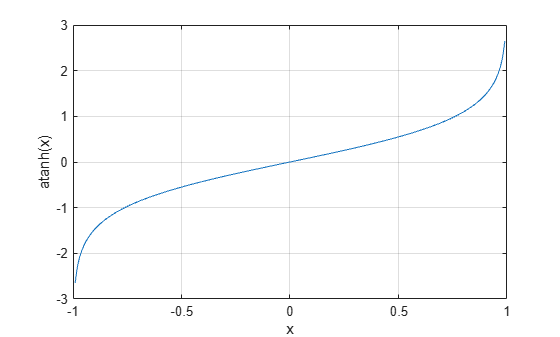atanh

Inverse hyperbolic tangent

Description

example

Y = atanh(X) returns the inverse hyperbolic tangent of the elements of X. The function accepts both real and complex inputs. All angles are in radians.

Examples

collapse all

Find the inverse hyperbolic tangent of the elements of vector X. The atanh function acts on X element-wise.

X = [2 -3 1+2i];
Y = atanh(X)
Y = 1×3 complex

0.5493 + 1.5708i  -0.3466 - 1.5708i   0.1733 + 1.1781i

Plot the inverse hyperbolic tangent function over the interval $-1.

x = -0.99:0.01:0.99;
plot(x,atanh(x))
grid on
xlabel('x')
ylabel('atanh(x)')Input Arguments

collapse all

Hyperbolic tangent of angle, specified as a scalar, vector, matrix, or multidimensional array. The atanh operation is element-wise when X is nonscalar.

Data Types: single | double
Complex Number Support: Yes

collapse all

Inverse Hyperbolic Tangent

For real values $x$ in the domain $-1, the inverse hyperbolic tangent satisfies

${\mathrm{tanh}}^{-1}\left(x\right)=\frac{1}{2}\mathrm{log}\left(\frac{1+x}{1-x}\right).$

For complex numbers $z=x+iy$ as well as real values in the regions $-\text{\hspace{0.17em}}\infty and $1, the call atanh(z) returns complex results.